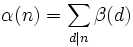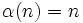# At most n elements of order dividing n implies every finite subgroup is cyclic

This article gives the statement and possibly, proof, of an implication relation between two group properties. That is, it states that every group satisfying the first group property (i.e., group with at most n elements of order dividing n) must also satisfy the second group property (i.e., group in which every finite subgroup is cyclic)
View all group property implications | View all group property non-implications
Get more facts about group with at most n elements of order dividing n|Get more facts about group in which every finite subgroup is cyclic

## Statement

### Property-theoretic statement

The group property of being a group with at most n elements of order dividing n is stronger than the group property of being a group in which every finite subgroup is cyclic.

### Statement with symbols

Suppose$G$ is a group, with identity element$1$, with the property that for any$n$, there are at most$n$ elements$x \in G$ satisfying$x^n = 1$. Then, every finite subgroup of$G$ is cyclic.

## Proof

Given: A group$G$ with the property that for every$n$, there are at most$n$ solutions to$x^n = 1$.

To prove: Every finite subgroup of$G$ is cyclic.

Proof: Let$\varphi(d)$ denote the Euler-phi function of$d$: in other words,$\varphi(d)$ is the number of elements that generate a given cyclic group of order$d$ (see fact (1) for more on this set of elements).

By fact (3), we then have, for any natural number$n$:$n = \sum_{d|n} \varphi(d)$

Let$A(d)$ be the set of all elements$x \in G$ such that$x^d = 1$ and$B(d)$ denote the set of all elements of order exactly$d$. Then, since$x^n = 1$ implies that the order of$x$ divides$n$, we get:$A(n) = \bigsqcup_{d|n} B(d)$.

Thus, if$\alpha(n), \beta(n)$ denote the cardinalities of$A(n), B(n)$ respectively, we get:$\alpha(n) = \sum_{d|n} \beta(d)$.

Next, we observe that$\beta(d) \in \{ 0, \varphi(d) \}$ for any$d$: If$\beta(d) = 0$, we are done. Otherwise, there exists a$g \in G$ of order exactly$d$. The cyclic subgroup$\langle g \rangle$ in$G$ is a cyclic group of order$d$. This has$d$ elements satisfying$x^d = 1$. Since there are at most$d$ elements in$G$ satisfying$x^d = 1$, we conclude that$A(d) = \langle g \rangle$. In this case, it is clear that$B(d)$ is precisely the set of generators of$\langle g \rangle$, which has size$\varphi(d)$. Thus,$\beta(d) = \varphi(d)$.

We now prove the claim by induction on order.

• Base case: The result is clearly true for a subgroup of order$1$.
• Induction step: Suppose$H$ is a finite subgroup of$G$ of order$n$. Note that every element of$H$ has order dividing$n$ (fact (4)), so$\alpha(n) \ge n$. But since there are at most$n$ elements of order$n$, we in fact have$\alpha(n) = n$ with$H = A(n)$. On the other hand, we have$\beta(d) \in \{ 0, \varphi(d) \}$ for all$d$, so$\beta(d) \le \varphi(d)$ for all divisors$d$ of$n$. Thus, we get:$n = \alpha(n) = \sum_{d|n} \beta(d) \le \sum_{d|n} \varphi(d) = n$.

Equality must hold throughout, forcing$\beta(d) = \varphi(d)$ for all$d | n$. In particular,$\beta(n) \ne 0$, so there exists an element of order exactly$n$. Further, since$H = A(n)$, this element must be in$H$, and we thus get that$H$ is the cyclic group of order$n$ on this element.